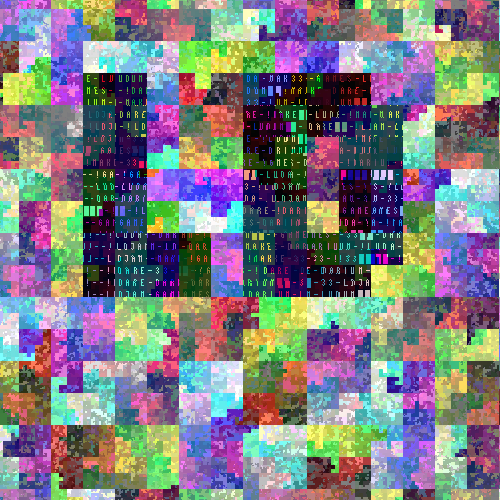## Ludum Dare 33 WallpaperWill Edwards over at ludumdare.com asked me how the wallpaper I made for LD was generated, so here goes.

I started with the code I had for pizhul – the actual image generation at 512x512 can be summarised in pseudocode as follows:

variation := 0 // 0, 1 or 2
size := 512
red, green, blue := 0
for pos := 0 to size * size do
x, y := hilbert(pos)
pixelRed[x, y] :=
(variation == 0 ? red : (variation == 1 ? green : blue)) << 16
pixelGreen[x, y] :=
((variation == 0 ? green : (variation == 1 ? blue : red)) >> 2) << 8
pixelBlue[x, y] :=
((variation == 0 ? blue : (variation == 1 ? red : green)) >> 4)
red := red + (50% of the time 3, otherwise 1)
red := red % 253
green := green + (50% of the time 3, otherwise 1)
green := green % 1007
blue := blue + (50% of the time 3, otherwise 1)
blue := bue % 4089
end for


Where variation changes the palette a bit, and hilbert(pos) is a call to a function that traces the path of a Hilbert curve.

For the wallpaper, the generation got a bit more complicated still. A grayscale effect was applied in a radial gradient around the LD letters, plus additional darkening further from the letters.

This line:

colour |= floor(random() > .9 ? (lrand = random()) * 7 : (lrand * 7)) * 0xF0F0F;


contributed a lot to the look of the wallpaper. lrand is a floating-point variable that acts as buffer for the complete noise that would otherwise be introduced with random(). Instead, the noise variable is only changed every 10th pixel (on average).

I wish I could publish the source code, but it is very messy and all over the place (different libraries), it runs as a very slow .swf that asks to save a file after some 10 seconds. After LD, I might clean it up and publish it.